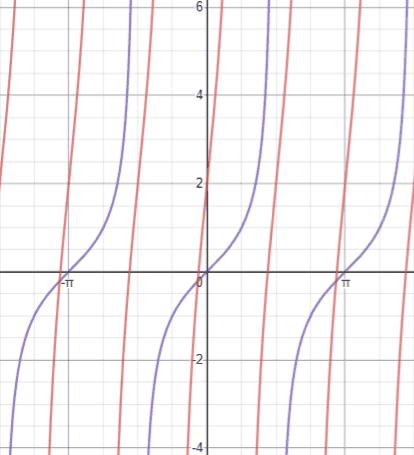# Tangent

Tangent, written as tan⁡(θ), is one of the six fundamental trigonometric functions.

## Tangent definitions

There are two main ways in which trigonometric functions are typically discussed: in terms of right triangles and in terms of the unit circle. The right-angled triangle definition of trigonometric functions is most often how they are introduced, followed by their definitions in terms of the unit circle.

### Right triangle definition

For a right triangle with one acute angle, θ, the tangent value of this angle is defined to be the ratio of the opposite side length to the adjacent side length.The sides of the right triangle are referenced as follows:

• Adjacent: the side next to θ that is not the hypotenuse
• Opposite: the side opposite θ.
• Hypotenuse: the longest side of the triangle opposite the right angle.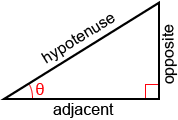The other two most commonly used trigonometric functions are cosine and sine, and they are defined as follows:Tangent is related to sine and cosine as:Example:

Find tan(⁡θ) for the right triangle below.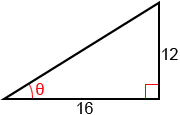We can also use the tangent function when solving real world problems involving right triangles.

Example:

Jack is standing 17 meters from the base of a tree. Given that the angle from Jack's feet to the top of the tree is 49°, what is the height of the tree, h? If the tree falls towards Jack, will it land on him?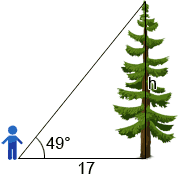Since we know the adjacent side and the angle, we can useto solve for the height of the tree.h = 17 × tan⁡(49°) ≈ 19.56

So, the height of the tree is 19.56 m. If Jack does not move, the tree will land on him if it falls in his direction, since 19.56 > 17.

### Unit circle definition

Trigonometric functions can also be defined with a unit circle. A unit circle is a circle of radius 1 centered at the origin. The right triangle definition of trigonometric functions allows for angles between 0° and 90° (0 andin radians). Using the unit circle definitions allows us to extend the domain of trigonometric functions to all real numbers. Refer to the figure below.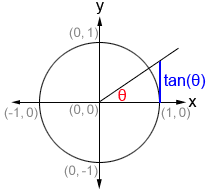On the unit circle, θ is the angle formed between the initial side of an angle along the x-axis and the terminal side of the angle formed by rotating the ray either clockwise or counterclockwise. On the unit circle, tan⁡(θ) is the length of the line segment formed by the intersection of the line x=1 and the ray formed by the terminal side of the angle as shown in blue in the figure above.

Unlike the definitions of trigonometric functions based on right triangles, this definition works for any angle, not just acute angles of right triangles, as long as it is within the domain of tan⁡(θ), which is undefined at odd multiples of 90° (). Thus, the domain of tan⁡(θ) is θ∈R,. The range of the tangent function is -∞<y<∞.

## Values of the tangent function

There are many methods that can be used to determine the value for tangent such as referencing a table of tangents, using a calculator, and approximating using the Taylor Series of tangent. In most practical cases, it is not necessary to compute a tangent value by hand, and a table, calculator, or some other reference will be provided.

### Tangent calculator

The following is a calculator to find out either the tangent value of an angle or the angle from the tangent value.

### Commonly used angles

While we can find tan⁡(θ) for any angle, there are some angles that are more frequently used in trigonometry. Below is a table of tangent values for commonly used angles in both radians and degrees.

Angle in degreesAngle in radiansTangent valueTangent value in decimals
000
15°0.268
30°0.577
45°11
60°1.732
75°3.732
90°UndefinedUndefined
180°π00
270°UndefinedUndefined
360°2π00

From these values, tangent can be determined as. Cosine has a value of 0 at 90° and a value of 1 at 0°. On the other hand, sine has a value of 1 at 90° and 0 at 0°. As a result, tangent is undefined whenever cos⁡(θ)=0, which occurs at odd multiples of 90° (), and is 0 whenever sin⁡(θ)=0, which occurs when θ is an integer multiple of 180° (π). The other commonly used angles are 30° (), 45° (), 60° () and their respective multiples. The cosine and sine values of these angles are worth memorizing in the context of trigonometry, since they are very commonly used, and can be used to determine values for tangent. Refer to the cosine and sine pages for their values.

Knowing the values of cosine, sine, and tangent for angles in the first quadrant allows us to determine their values for corresponding angles in the rest of the quadrants in the coordinate plane through the use of reference angles.

### Reference angles

A reference angle is an acute angle (<90°) that can be used to represent an angle of any measure. Any angle in the coordinate plane has a reference angle that is between 0° and 90°. It is always the smallest angle (with reference to the x-axis) that can be made from the terminal side of an angle. The figure below shows an angle θ and its reference angle θ'.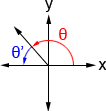Because θ' is the reference angle of θ, both tan⁡(θ) and tan⁡(θ') have the same value. For example, 30° is the reference angle of 150°, and their tangents both have a magnitude of, albeit they have different signs, since tangent is positive in quadrant I but negative in quadrant II. Because all angles have a reference angle, we really only need to know the values of tan⁡(θ) (as well as those of other trigonometric functions) in quadrant I. All other corresponding angles will have values of the same magnitude, and we just need to pay attention to their signs based on the quadrant that the terminal side of the angle lies in. Below is a table showing the signs of cosine, sine, and tangent in each quadrant.

 Tangent Sine Cosine Quadrant I + + + Quadrant II - + - Quadrant III + - - Quadrant IV - - +

Once we determine the reference angle, we can determine the value of the trigonometric functions in any of the other quadrants by applying the appropriate sign to their value for the reference angle. The following steps can be used to find the reference angle of a given angle, θ:

1. Subtract 360° or 2π from the angle as many times as necessary (the angle needs to be between 0° and 360°, or 0 and 2π). If the resulting angle is between 0° and 90°, this is the reference angle.
2. Determine what quadrant the terminal side of the angle lies in (the initial side of the angle is along the positive x-axis)
3. Depending what quadrant the terminal side of the angle lies in, use the equations in the table below to find the reference angle. In quadrant I, θ'=θ.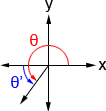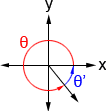θ'= 180° - θ θ'= θ - 180° θ'= 360° - θ

Example:

Find tan⁡(240°).

1. θ is already between 0° and 360°
2. 240° lies in quadrant III
3. 240° - 180° = 60°, so the reference angle is 60°

tan⁡(60°)=. 240° is in quadrant III where tangent is positive, so: tan⁡(240°)=tan⁡(60°)=Example:

Find tan⁡(690°).

1. 690° - 360° = 330°
2. 330° lies in quadrant IV
3. 360° - 330° = 30°

tan⁡(30°) =. 330° is in quadrant IV where tangent is negative, so:

tan⁡(330°) = -tan⁡(30°) =## Properties of the tangent function

Below are a number of properties of the tangent function that may be helpful to know when working with trigonometric functions.

### Tangent is a cofunction of cotangent

A cofunction is a function in which f(A) = g(B) given that A and B are complementary angles. In the context of tangent and cotangent,

tan⁡(θ) = cot⁡(90° - θ)

cot⁡(θ) = tan⁡(90° - θ)

Example:

tan⁡(30°) = cot⁡(90° - 30°)

tan⁡(30°) = cot⁡(60°)Referencing the unit circle shown above, the fact that, and, we can see that:

tan⁡(30°) = cot⁡(60°) =### Tangent is an odd function

An odd function is a function in which -f(x)=f(-x). It has symmetry about the origin. Thus,

-tan⁡(θ) = tan⁡(-θ)

Example:

-tan⁡(30°) = tan⁡(-30°)

-tan⁡(30°) = tan⁡(330°)Referencing the unit circle or a table, we can find that tan⁡(30°)=. tan⁡(-30°) is equivalent to tan⁡(330°), which we determine has a value of. Thus, -tan⁡(30°) = tan⁡(330°) =.

### Tangent is a periodic function

A periodic function is a function, f, in which some positive value, p, exists such that

f(x+p) = f(x)

for all x in the domain of f, p is the smallest positive number for which f is periodic, and is referred to as the period of f. The period of the tangent function is π, and it has vertical asymptotes at odd multiples of. We can write this as:

tan⁡(θ+π) = tan⁡(θ)

To account for multiple full rotations, this can also be written as

tan⁡(θ+nπ) = tan⁡(θ)

where n is an integer.

Unlike sine and cosine, which are continuous functions, each period of tangent is separated by vertical asymptotes.

Example:tan⁡(405°) = tan(45° + 2×180°) = tan(45°) = 1

## Graph of the tangent function

The graph of tangent is periodic, meaning that it repeats itself indefinitely. Unlike sine and cosine however, tangent has asymptotes separating each of its periods. The domain of the tangent function is all real numbers except whenever cos⁡(θ)=0, where the tangent function is undefined. This occurs whenever. This can be written as θ∈R,. Below is a graph of y=tan⁡(x) showing 3 periods of tangent.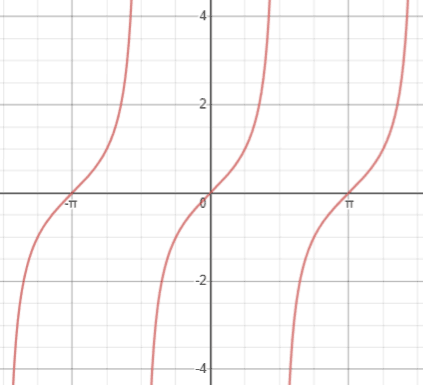In this graph, we can see that y=tan⁡(x) exhibits symmetry about the origin. Reflecting the graph across the origin produces the same graph. This confirms that tangent is an odd function, since -tan⁡(x)=tan(-x).

### General tangent equation

The general form of the tangent function is

y = A·tan(B(x - C)) + D

where A, B, C, and D are constants. To be able to graph a tangent equation in general form, we need to first understand how each of the constants affects the original graph of y=tan⁡(x), as shown above. To apply anything written below, the equation must be in the form specified above; be careful with signs.

A—the amplitude of the function; typically, this is measured as the height from the center of the graph to a maximum or minimum, as in sin⁡(x) or cos⁡(x). Since y=tan⁡(x) has a range of (-∞,∞) and has no maxima or minima, rather than increasing the height of the maxima or minima, A stretches the graph of y=tan⁡(x); a larger A makes the graph approach its asymptotes more quickly, while a smaller A (<1) makes the graph approach its asymptotes more slowly. This is sometimes referred to as how steep or shallow the graph is, respectively.

Compared to y=tan⁡(x), shown in purple below, the function y=5tan⁡(x) (red) approaches its asymptotes more steeply.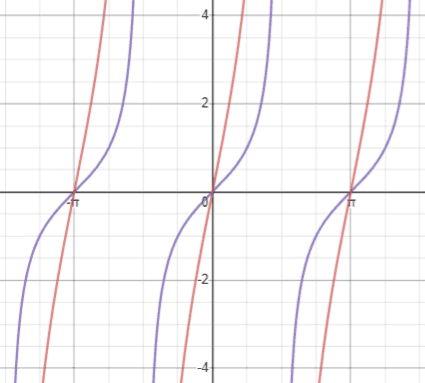B—used to determine the period of the function; the period of a function is the distance from peak to peak (or any point on the graph to the next matching point) and can be found as. In y=tan⁡(x) the period is π. We can confirm this by looking at the tangent graph. Referencing the figure above, we can see that each period of tangent is bounded by vertical asymptotes, and each vertical asymptote is separated by an interval of π, so the period of the tangent function is π.

Compared to y=tan⁡(x), shown in purple below, which has a period of π, y=tan⁡(2x) (red) has a period of. This means that the graph repeats itself everyrather than every π.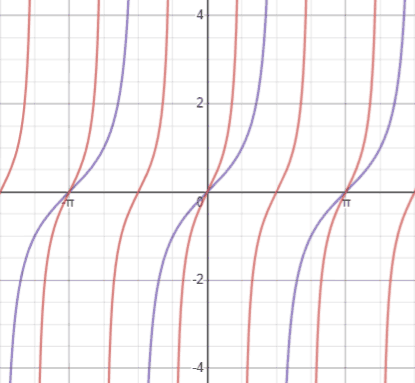C—the phase shift of the function; phase shift determines how the function is shifted horizontally. If C is negative, the function shifts to the left. If C is positive the function shifts to the right. Be wary of the sign; if we have the equationthen C isnot, because this equation in standard form is. Thus, we would shift the graphunits to the left.

The figure below shows y=tan⁡(x) (purple) and(red). Using the zero of y=tan⁡(x) at (0, 0) as a reference, we can see that the same zero inhas been shifted to (, 0).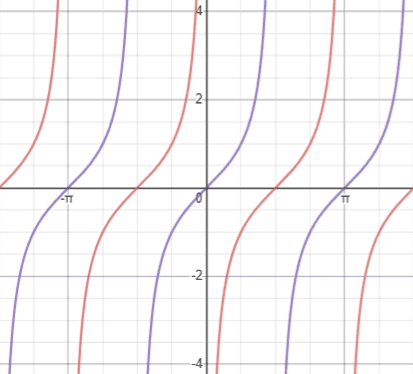D—the vertical shift of the function; if D is positive, the graph shifts up D units, and if it is negative, the graph shifts down.

Compared to y=tan⁡(x), shown in purple below, which is centered at the x-axis (y=0), y=tan⁡(x)+2 (red) is centered at the line y=2 (blue).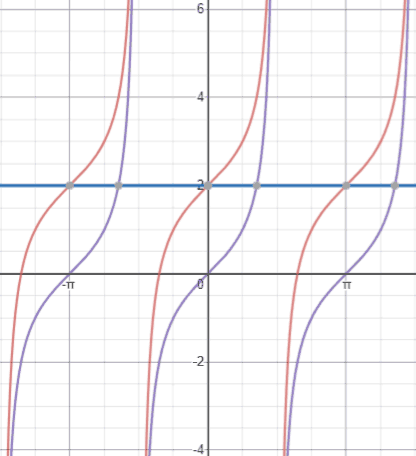Putting together all the examples above, the figure below shows the graph of(red) compared to that of y=tan⁡(x) (purple).